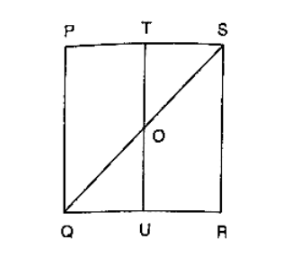# In figure, PQRS is a square and T and U are, respectively, the midpoints of PS and QR.

Question:

In figure, PQRS is a square and T and U are, respectively, the midpoints of PS and QR. Find the area of ΔOTS if PQ = 8 cm.Solution:

From the figure,

T and U are mid points of PS and QR respectively

∴ TU ∥ PQ

⇒ TO ∥ PQ

Thus, in ΔPQS, T   is the midpoint of PS and TO ∥ PQ

∴ TO = (1/2) PQ = 4 cm

Also, TS = (1/2) PS = 4 cm

$\therefore \operatorname{ar}(\Delta \mathrm{OTS})=(1 / 2)(\mathrm{TO} \times \mathrm{TS})=(1 / 2)(4 \times 4) \mathrm{cm}^{2}=8 \mathrm{~cm}^{2}$